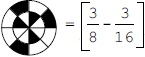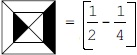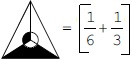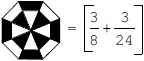# User Forum

Subject :IMO    Class : Class 4

Which of the following options is correct with respect to fraction of unshaded parts of the figures?

ABCDClass : Class 5

## Ans 2:

Class : Class 6
can someone please explain this:Answer is D Unshaded part Given options A (10/16)3/8 - 3/16 = 3/16 B (6/8)Â½ - Â¼ = 1/4 C (4/6)1/6 1/3 = 3/6 = 1/2 D (8/16)3/8 3/24 = 12/24 = Â½

## Ans 3:

Class : Class 6
Answer is D Unshaded part Given options A (10/16)3/8 - 3/16 = 3/16 B (6/8)Â½ - Â¼ = 1/4 C (4/6)1/6 1/3 = 3/6 = 1/2 D (8/16)3/8 3/24 = 12/24 = Â½

Class : Class 6
D

Class : Class 6
How?